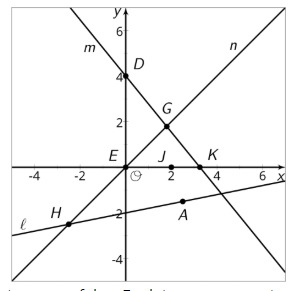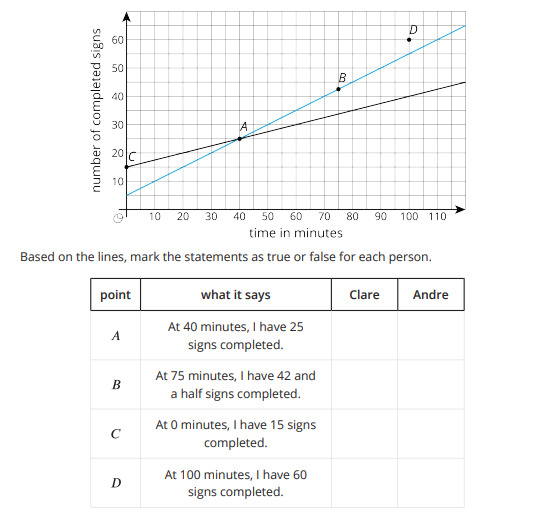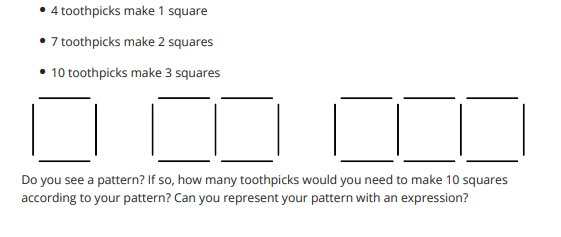PSABM6WD - Student Facing Task---Acc7.5 Lesson 11 More Solutions to Linear Equations (8.EE.C, 8.EE.C.8, 8.EE.C.8.a)
Part A)

For each equation choose a value for x and then solve to find the corresponding y value that makes that equation true.Part B)

For each equation choose a value for x and then solve to find the corresponding y value that makes that equation true.Part C)

For each equation choose a value for x and then solve to find the corresponding y value that makes that equation true.Part A)

Here are graphs representing three linear relationships. These relationships could also be represented with equations.For each statement below, decide if it is true or false. Explain your reasoning.

(4,0) is a solution of the equation for line m.

Select one:
Part B)

Part C)

For each statement below, decide if it is true or false. Explain your reasoning.

The coordinates of the point G make both the equation for line m and the equation for line n true.

Select one:
Part D)

Part E)

For each statement below, decide if it is true or false. Explain your reasoning.

x=0 is a solution of the equation for line n.

Select one:
Part F)

Part G)

For each statement below, decide if it is true or false. Explain your reasoning.

(2,0) makes both the equation for line m and the equation for line n true.

Select one:
Part H)

Part I)

For each statement below, decide if it is true or false. Explain your reasoning.

There is no solution for the equation for line ℓ that has y=0.

Select one:
Part J)

Part K)

For each statement below, decide if it is true or false. Explain your reasoning.

The coordinates of point H are solutions to the equation for line .

Select one:
Part L)

Part M)

For each statement below, decide if it is true or false. Explain your reasoning.

There are exactly two solutions of the equation for line .

Select one:
Part N)

Part O)

For each statement below, decide if it is true or false. Explain your reasoning.

There is a point whose coordinates make the equations of all three lines true.

Select one:
Part P)

Part A)

Consider the equation ax + by = c, where a, b, and c are positive numbers.

Find the coordinates of the x- and y-intercepts of the graph of the equation.

Part B)

Find the slope of the graph.

Part A)

Clare and Andre are making signs for all the lockers as part of the decorations for the upcoming spirit week. Yesterday, Andre made 15 signs and Clare made 5 signs. Today, they need to make more signs. Each person's progress today is shown in the coordinate plane.For point A is the statement for Clare True or False?

Select one:
Part B)

Clare and Andre are making signs for all the lockers as part of the decorations for the upcoming spirit week. Yesterday, Andre made 15 signs and Clare made 5 signs. Today, they need to make more signs. Each person's progress today is shown in the coordinate plane.For point A is the statement for Andre True or False?

Select one:
Part C)

Clare and Andre are making signs for all the lockers as part of the decorations for the upcoming spirit week. Yesterday, Andre made 15 signs and Clare made 5 signs. Today, they need to make more signs. Each person's progress today is shown in the coordinate plane.For point B is the statement for Clare True or False?

Select one:
Part D)

Clare and Andre are making signs for all the lockers as part of the decorations for the upcoming spirit week. Yesterday, Andre made 15 signs and Clare made 5 signs. Today, they need to make more signs. Each person's progress today is shown in the coordinate plane.For point B is the statement for Andre True or False?

Select one:
Part E)

Clare and Andre are making signs for all the lockers as part of the decorations for the upcoming spirit week. Yesterday, Andre made 15 signs and Clare made 5 signs. Today, they need to make more signs. Each person's progress today is shown in the coordinate plane.For point C is the statement for Clare True or False?

Type your answer below as a number (example: 5, 3.1, 4 1/2, or 3/2):
Part F)

Clare and Andre are making signs for all the lockers as part of the decorations for the upcoming spirit week. Yesterday, Andre made 15 signs and Clare made 5 signs. Today, they need to make more signs. Each person's progress today is shown in the coordinate plane.For point C is the statement for Andre True or False?

Type your answer below as a number (example: 5, 3.1, 4 1/2, or 3/2):
Part G)

Clare and Andre are making signs for all the lockers as part of the decorations for the upcoming spirit week. Yesterday, Andre made 15 signs and Clare made 5 signs. Today, they need to make more signs. Each person's progress today is shown in the coordinate plane.For point D is the statement for Clare True or False?

Type your answer below as a number (example: 5, 3.1, 4 1/2, or 3/2):
Part H)

Clare and Andre are making signs for all the lockers as part of the decorations for the upcoming spirit week. Yesterday, Andre made 15 signs and Clare made 5 signs. Today, they need to make more signs. Each person's progress today is shown in the coordinate plane.For point D is the statement for Andre True or False?

Select one: